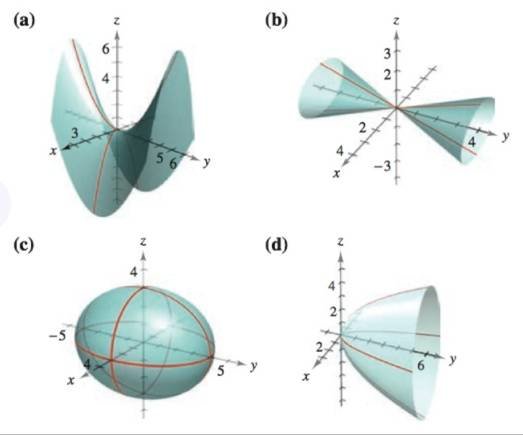Chapter 11.6, Problem 4E

Chapter
Section
Textbook Problem

# Matching In Exercises 5-10, match the equation with its graph. [The graphs are labeled (a), (b), (c), (d), (e), and (F).]y 2 = 4 x 2 + 9 z 2

To determine
Equation y2=4x2+9z2 is given. Find the graph that matches the given equation.

Explanation

Given:

The provided equation is

y2=4x2+9z2

Explanation:

Equation y2=4x2+9z2 is given.

simplify,

4x2y2+9z2=0

Further, x21/4y2+z21/9=0 …… (1)

As we know elliptic cone centered o

### Still sussing out bartleby?

Check out a sample textbook solution.

See a sample solution

#### The Solution to Your Study Problems

Bartleby provides explanations to thousands of textbook problems written by our experts, many with advanced degrees!

Get Started